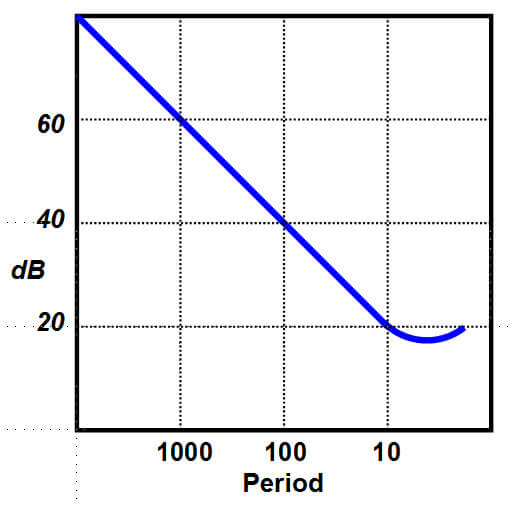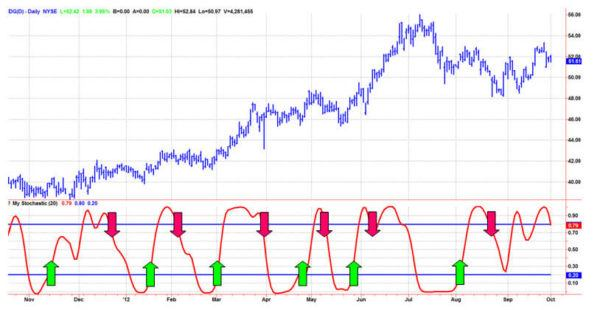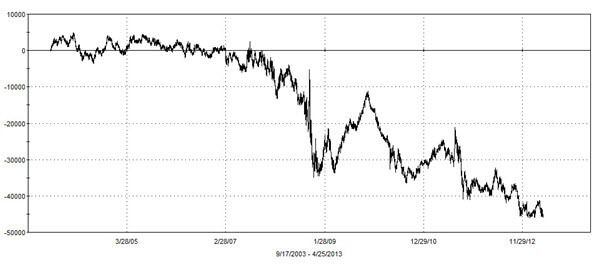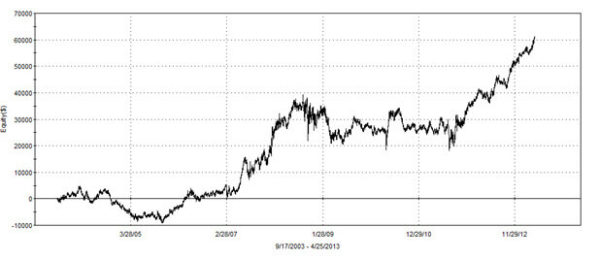# Predictive Indicators### INTRODUCTION

Technical traders understand that indicators need to smooth market data to be useful, and that smoothing introduces lag as an unwanted side effect. We also know that the market is fractal; a daily interval chart looks just like a weekly, monthly, or intraday chart. What may not be quite as obvious is that as the time interval along the x-axis increases, the high-to-low price that swings along the y-axis also increases, roughly in proportion. This “spectral dilatation” phenomena causes an undesirable distortion, one that has either not been recognized or has been largely ignored by indicator developers and market technicians.

So, we have two challenges to address. 1) How to best smooth the data; and 2) how the fractal nature of the market distorts indicator interpretation.

To address the smoothing problems I will show you a stunning new filter from my aerospace analog filter design experience translated to a digital filter useful for traders. To address the mitigating effects of the fractal structure of the market I will show you the effects of a new filter seldom used in trading. By removing the noise and the indicator distortion I will then show you the core concept of getting consistent short term trading winners.

### MARKET DATA STRUCTURE

Picture a chart using daily data. Next, picture a chart using weekly data. They basically look the same when the scales are removed. This is often called the fractal nature of the market. Since the charts look the same, and since the time scales are different by a factor of 5, it must be concluded that the cyclic swings are five times greater when using weekly data. Therefore, the cycle amplitudes in market data are in direct proportion to their wavelength for the fractal dimensions to hold.

There have been many academic studies about the market data structure. Figure 1 captures the significant factors found in these studies. The horizontal axis is in terms of frequency, so that a frequency of 10-1 means a cycle having a 10-bar period and a frequency of 10-2 means a cycle having a 100-bar period, etc. The vertical scale is the power in the wave.

Aliasing noise has the largest amplitude at the higher frequencies (i.e. the shortest cycle periods). Since Aliasing Noise is larger than signals at these cycle periods, noise swamps the information in the signal, and the only thing to do is to avoid trying to use signals that fall within the range of these cycle periods. Aliasing Noise is also as large or larger than signals at longer cycle periods and therefore it must be removed by smoothing to recover the signal.

Starting at periods somewhat shorter than a 10-bar cycle, a longer wave has more power in it than a shorter wave, which means the wave amplitude is larger for the longer wave. I call the shape of this Wave Amplitude versus frequency Spectral Dilation.

Spectral Dilation increases at the rate of approximately 6 dB per octave. This means that each time the cycle period is doubled, the wave amplitude in that longer wave is also doubled. It works the other way too. That is, every time the cycle period is halved the wave amplitude is also halved.

Although I knew about Spectral Dilation I was shocked to find that the phenomenon exists all the way down to shorter cycle periods – even below a 10-bar cycle period. At this point Spectral Dilation intercepts the Aliasing Noise resulting from using sampled data. If we just want to eliminate Aliasing Noise, we do not need a smoothing filter longer than a 10-bar cycle period. In fact, using a smoothing filter longer than 10 bars attenuates the data we are trying to use in trading.Figure 1. Market Data Contains Both Aliasing Noise and Spectral Dilation. Aliasing Noise Swamps the Signal Where the Cycle Period is Less than 10 Bars

It is also worthy of note that Aliasing Noise scales with the sampling frequency, and so it is a good rule of thumb that Aliasing Noise intercepts the signal amplitude at about a 10-bar cycle period regardless of the time frame you are trading.

### SMOOTHING FILTERS

Most traders use a moving average for smoothing. The problem is that a moving average is just not a very efficient filter. In order to get the amount of smoothing desired, the moving average length must be made longer. But making the average length longer also causes more lag, with the result that the smoothed trading signals are not timely for a good trade. My SuperSmoother filter is much more efficient. I created the SuperSmoother digital filter by converting an analog filter having real inductors and capacitors into the digital domain and then minimizing the lag of the converted filter. I have compared a 10-bar Exponential Moving Average (EMA) with my SuperSmoother filter set to a critical period of 10 bars for an apples-to-apples comparison in Figure 2. The horizontal scale is in terms of frequency, so that 0.1 corresponds to a 10-bar cycle period and .5 corresponds to a 2-bar cycle period. 0.5 is called the Nyquist frequency, and is the highest possible frequency component using sampled data.

The EMA only reduces the amplitude at the Nyquist frequency by 13 dB. On the other hand, the SuperSmoother filter theoretically completely eliminates components at the Nyquist Frequency. The added benefit is that the SuperSmoother filter has significantly less lag than the EMA.Figure 2. An EMA Has Only Modest Noise Attenuation While a SuperSmoother Virtually Eliminates Aliasing Noise

Code Listing 1 gives the EasyLanguage code to compute the Super Smoother filter. The a1 and b1 variables are computed for the 10-bar critical period. These are then used to compute the c1, c2, and, c3 coefficients of the filter. If the reader is translating to another computer language, please note that the trigonometric arguments are in degrees whereas they are in radians in most other languages. Also please note that the notation [N] is the value of that variable “N” bars ago.

Code Listing 1.  EasyLanguage Code to Compute the SuperSmoother Filter
{SuperSmoother filter

Vars:  a1(0), b1(0), c1(0), c2(0), c3(0), Filt(0);

a1 = expvalue(-1.414*3.14159 / 10);

b1 = 2*a1*Cosine(1.414*180 / 10);

c2 = b1;

c3 = -a1*a1;

c1 = 1 - c2 - c3;

Filt = c1*(Close + Close) / 2 + c2*Filt + c3*Filt;

Plot1(Filt);

Plot2(0);

Having solved the problem of dealing with Aliasing Noise, we can now turn our attention to Spectral Dilation.

### SPECTRAL DILATION

Most trading oscillators and “detrenders” perform basically the same way as a 1-pole HighPass filter because they only have one difference term in their calculation. For example, the difference term of a Stochastic Indicator subtracts the lowest closing price over the length of the indicator from the current closing price. The result of performing as a 1-pole HighPass filter is that the attenuation scales as 6 dB per octave for frequency components smaller than the critical frequency.

But if Spectral Dilation is increasing the amplitude at the rate of 6 dB per octave and the oscillator is reducing the amplitude due to filtering at the rate of 6 dB per octave, the net result is that the longer period waves are not filtered out. The only effect of the single pole filter (or conventional indicator) is to flatten and equalize the Spectral Density. Figure 3 shows the output of a single pole HighPass filter that is smoothed with a SuperSmoother filter.Figure 3. A Single Pole HighPass Filter (or Oscillator) Does Not Remove Low Frequency Components, Resulting in the Output Failing to Have a Zero Mean

If the high pass filter complexity is increased to a 2-pole HighPass filter, then the filter response increases to 12 dB per octave, enabling the effects of Spectral Dilation to be removed. The result of using the 2-pole HighPass filter is compared to the response of the original 1-pole HighPass filter in Figure 4.

The original 1-pole HighPass filter output is shown as the red dashed line and the 2-pole HighPass filter output shows that the effects of Spectral Dilation have been removed. Now the output has a nominally zero mean, allowing for a more accurate assessment of the turning points without the distortion of the long period offsets. In addition, the waveform generally has less lag when longer wave components are removed.Figure 4. A Two Pole HighPass Filter Removes the Effects of Spectral Dilation. This Gives the Oscillator a Zero Mean to Accurately Assess Turning Points and Generally Reduces Indicator Lag.

The combination of a 2-pole HighPass filter and a SuperSmoother filter can be used to precondition data before any additional calculations are performed. Since this filter combination limits the range of cycle periods in the output, it is called a “Roofing Filter” because it provides a roof over further calculations. The EasyLanguage code to compute the Roofing filter is given in Code Listing 2.

Code Listing 2.  EasyLanguage Code to Compute the Roofing Filter
{Roofing filter

Vars: alpha1(0), HP(0), a1(0), b1(0), c1(0), c2(0), c3(0), Filt(0), Filt2(0);

//Highpass filter cyclic components whose periods are shorter than 48 bars
alpha1 = (Cosine(.707*360 / 48) + Sine (.707*360 / 48) - 1) / Cosine(.707*360 / 48); HP = (1 - alpha1 / 2)*(1 - alpha1 / 2)*(Close - 2*Close + Close) + 2*(1 - alpha1)*HP - (1 - alpha1)*(1 - alpha1)*HP;

//Smooth with a Super Smoother Filter
a1 = expvalue(-1.414*3.14159 / 10);
b1 = 2*a1*Cosine(1.414*180 / 10);
c2 = b1;
c3 = -a1*a1;
c1 = 1 - c2 - c3;
Filt = c1*(HP + HP) / 2 + c2*Filt + c3*Filt;

Plot1(Filt);
Plot2(0);

In Code Listing 2, the variable alpha1 is computed for the HighPass critical period of 48 bars. The HighPass filter will pass all spectral components having periods less than 48 bars. The SuperSmoother filter passes all spectral components having period greater than 10 bars. The end result of the Roofing filter is that cyclic components having periods between 10 bars and 48 bars will be passed from the input to the output. If the reader is translating to another computer language, please note that the trigonometric arguments are in degrees whereas they are in radians in most other languages. Also please note that the notation [N] is the value of that variable “N” bars ago.

The impact of using the Roofing filter before computing a Stochastic is shown in Figure5. Clearly, the conventional Stochastic tends to hang near the upper bound when the prices are in an uptrend. Since this characteristic is caused by Spectral Dilation, the tired old explanations of how to use %K, %D, and when to enter a short position at the end of a trend are just plain silly. When the Roofing filter precedes the Stochastic, as shown in the second subgraph of Figure 5, the longer period waves have been removed and the swing waves are obvious by inspection.Figure 5. A Roofing Filter Preceding a Stochastic Removes Spectral Dilation Distortion

The Stochastic calculation is simply the current close minus the lowest close over the calculation period, normalized to the highest close minus the lowest close. Although this calculation is trivial, I have included the complete indicator in Code Listing 3 for the convenience of readers who wish to duplicate it. As described above, I use the output of the Roofing filter for the Stochastic calculation rather than the closing price. Also note that I use a SuperSmoother filter rather than an exponential moving average to smooth the result of the calculations. I call the indicator, “My Stochastic” to avoid confusion with the conventional Stochastic indicator.

Code Listing 3. EasyLanguage Code to Compute My Stochastic
{My Stochastic Indicator

Inputs: Length(20);

Vars: alpha1(0), HP(0), a1(0), b1(0), c1(0), c2(0), c3(0), Filt(0),
HighestC(0), LowestC(0), count(0), Stoc(0), MyStochastic(0);

//Highpass filter cyclic components whose periods are shorter than 48 bars
alpha1 = (Cosine(.707*360 / 48) + Sine (.707*360 / 48) - 1) / Cosine(.707*360 / 48);
HP = (1 - alpha1 / 2)*(1 - alpha1 / 2)*(Close - 2*Close + Close) + 2*(1 - alpha1)*HP - (1 - alpha1)*(1 - alpha1)*HP;

//Smooth with a Super Smoother Filter
a1 = expvalue(-1.414*3.14159 / 10);
b1 = 2*a1*Cosine(1.414*180 / 10);
c2 = b1;
c3 = -a1*a1;
c1 = 1 - c2 - c3;
Filt = c1*(HP + HP) / 2 + c2*Filt + c3*Filt;

HighestC = Filt;
LowestC = Filt;
For count = 0 to Length - 1 Begin
If Filt[count] > HighestC then HighestC = Filt[count];
If Filt[count] < LowestC then LowestC = Filt[count];
End;
Stoc = (Filt - LowestC) / (HighestC - LowestC);
MyStochastic = c1*(Stoc + Stoc) / 2 + c2*MyStochastic + c3*MyStochastic;

Plot1(MyStochastic);
Plot2(.8);
Plot6(.2);

Having successfully dealt with the Aliasing Noise issues as well as Spectral Dilation in the price data, we now have the tools to address trading strategies.

Conventional wisdom dictates that a long position trade be entered after the oscillator indicator has reached a minimum and turns up. The thinking here is that the indicator is so unreliable that confirmation of the price movement must be obtained before the trade is made. The conventional trading rules are depicted in Figure 6. Buy signals are created when the indicator crosses over the 20% level. Sell Short (or Sell to Exit) signals are created when the indicator crosses under the 80% level.Figure 6. Conventional Wisdom is to Buy When the Indicator Crosses Above 20% and To Sell Short when the Indicator Crosses below 80%

We can test this hypothesis. I am going to describe a trading strategy using the Stochastic preceded by a Roofing filter of Code Listing 3. I want to emphasize this is not an explicit trading system, it is only a demonstration of a strategy. The strategy using confirmation is to buy when the indicator has reached a minimum value and then crosses over the 20% level. Also confirmation is used to sell short when the maximum value has been reached and then crosses below the 80% level. The equity curve applying this simple rule to the Stochastic oscillator on 10 years of S&P daily Futures data is shown in Figure 7. Clearly, one would not want to trade this rule in real life.Figure 7. Consistent Losses are Incurred When Trading the Stochastic Oscillator Using the Conventional Rule of Waiting for Confirmation

On the other hand, if we have removed the effects of Aliasing Noise as well as Spectral Dilation from the indicator we can conquer lag by anticipating the cyclic turning points. In other words, we have established a predictive and forecasting indicator. For example, to anticipate the turning point using the Roofing filter and Stochastic indicator, we would take a long position when the indicator crosses below the 20% level, BEFORE the indicator reaches its minimum value. Accordingly, we would take a short position when the indicator crosses above its 80% level,  BEFORE the indicator reaches its maximum value. These trading rules are depicted in Figure 7.Figure 8. Predictive Indicators Enable You to Buy When the Indicator Crosses Below 20% and To Sell Short when the Indicator Crosses Above 80%

Doing just this, with no other rules, we obtain the equity curve shown in Figure 9 when trading the S&P daily Futures over the last 10 years. Clearly, anticipating the turning points works and therefore the correctly filtered indicator is predictive! I want to emphasize again, that this is not a complete trading system. Rather, I have just demonstrated the efficacy of anticipating the indicator turning points.Figure 9. Consistent Winners Occur When Trading the Stochastic Oscillator by Anticipating the Indicator Turning Points

### CONCLUSIONS

I have shown that the information at very short cycle periods is swamped by Aliasing Noise, and therefore the only solution is to avoid these cycle periods in your analyses. Since Aliasing Noise scales with the sample frequency, periods shorter than about 10 bars should be avoided regardless of the time scale on which you are trading. Aliasing Noise is also as large as signals at longer cycle periods, and therefore should be removed by filtering. The SuperSmoother filter is vastly superior to moving averages for removing Aliasing Noise as well as other general smoothing applications.

The SuperSmoother filter can be combined with a 2-pole HighPass filter to create a Roofing filter. The Roofing filter determines the segment of the frequency spectrum to be used for analysis. The Roofing filter removes the effect of Spectral Dilation and helps create oscillators having a nearly zero mean.

Smoothed indicators having a symmetrical swing about a nominal zero mean can be used to create reliable predictive indicators. Most technical analysis indicators merely document what has happened in history. Who needs that? The purpose of indictors is to make a statistically reliable prediction of what the future holds so that a signal can be converted to a profitable trade.

— By John Ehlers of MESA Software

### About the Author John Ehlers

•Peter Maier says:

First of thanks for this article. I think it can really come in handy, being able to smooth the data in such an simple, jet effective way.
But I have a question. You state that:
“I created the SuperSmoother digital filter by converting an analog filter having real inductors and capacitors into the digital domain and then minimizing the lag of the converted filter.”

What is the name of the filter you originally used? Because it might be helpful in an analog project.
Thanks.